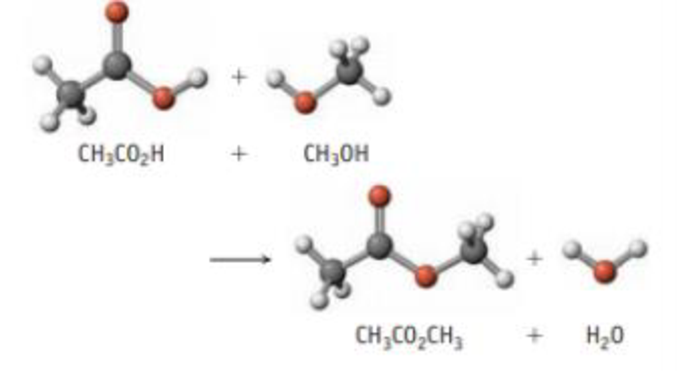Chapter 14, Problem 98SCQ

Chapter
Section
Textbook Problem

Isotopes are often used as “tracers” to follow an atom through a chemical reaction, and the following is an example. Acetic acid reacts with methanol.Explain how you could use the isotope 18O to show whether the oxygen atom in the water comes from the —OH of CH3CO2H or the —OH of CH3OH.

Interpretation Introduction

Interpretation: Considering the given chemical reaction it should be explained that how O18 isotope is used to prove that oxygen atom in H2O comes from methanol or from acetic acid.

Concept introduction:

Isotope: Two atoms are isotopes if they have equal number of protons but with different number of neutrons that is two atoms with same atom number but with different mass number are considered as isotopes.

One of the important applications of isotope is that they are used in isotopic labelling that is they are used as tracers in order to analysis the compound and to find out from which reactant molecule it forms.

Explanation

Using O18 labelled alcohol that is the oxygen atom present in alcohol is O18 isotope and finally it is found that the water formed does not contain O18 isotope

Still sussing out bartleby?

Check out a sample textbook solution.

See a sample solution

The Solution to Your Study Problems

Bartleby provides explanations to thousands of textbook problems written by our experts, many with advanced degrees!

Get Started